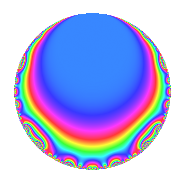# Properties

 Label 276.1.h.cLevel 276 Weight 1 Character orbit 276.h Analytic conductor 0.138 Analytic rank 0 Dimension 2 Projective image $$D_{6}$$ CM disc. -23 Inner twists 4

# Related objects

## Newspace parameters

 Level: $$N$$ = $$276 = 2^{2} \cdot 3 \cdot 23$$ Weight: $$k$$ = $$1$$ Character orbit: $$[\chi]$$ = 276.h (of order $$2$$ and degree $$1$$)

## Newform invariants

 Self dual: No Analytic conductor: $$0.137741943487$$ Analytic rank: $$0$$ Dimension: $$2$$ Coefficient field: $$\Q(\zeta_{6})$$ Coefficient ring: $$\Z[a_1, a_2]$$ Coefficient ring index: $$1$$ Projective image $$D_{6}$$ Projective field Galois closure of 6.2.914112.1

## $q$-expansion

The $$q$$-expansion and trace form are shown below.

 $$f(q)$$ $$=$$ $$q + \zeta_{6}^{2} q^{2} -\zeta_{6}^{2} q^{3} -\zeta_{6} q^{4} + \zeta_{6} q^{6} + q^{8} -\zeta_{6} q^{9} +O(q^{10})$$ $$q + \zeta_{6}^{2} q^{2} -\zeta_{6}^{2} q^{3} -\zeta_{6} q^{4} + \zeta_{6} q^{6} + q^{8} -\zeta_{6} q^{9} - q^{12} + q^{13} + \zeta_{6}^{2} q^{16} + q^{18} - q^{23} -\zeta_{6}^{2} q^{24} - q^{25} + \zeta_{6}^{2} q^{26} - q^{27} + ( \zeta_{6} + \zeta_{6}^{2} ) q^{29} + ( \zeta_{6} + \zeta_{6}^{2} ) q^{31} -\zeta_{6} q^{32} + \zeta_{6}^{2} q^{36} -\zeta_{6}^{2} q^{39} + ( -\zeta_{6} - \zeta_{6}^{2} ) q^{41} -\zeta_{6}^{2} q^{46} - q^{47} + \zeta_{6} q^{48} - q^{49} -\zeta_{6}^{2} q^{50} -\zeta_{6} q^{52} -\zeta_{6}^{2} q^{54} + ( -1 - \zeta_{6} ) q^{58} + 2 q^{59} + ( -1 - \zeta_{6} ) q^{62} + q^{64} + \zeta_{6}^{2} q^{69} + q^{71} -\zeta_{6} q^{72} - q^{73} + \zeta_{6}^{2} q^{75} + \zeta_{6} q^{78} + \zeta_{6}^{2} q^{81} + ( 1 + \zeta_{6} ) q^{82} + ( 1 + \zeta_{6} ) q^{87} + \zeta_{6} q^{92} + ( 1 + \zeta_{6} ) q^{93} -\zeta_{6}^{2} q^{94} - q^{96} -\zeta_{6}^{2} q^{98} +O(q^{100})$$ $$\operatorname{Tr}(f)(q)$$ $$=$$ $$2q - q^{2} + q^{3} - q^{4} + q^{6} + 2q^{8} - q^{9} + O(q^{10})$$ $$2q - q^{2} + q^{3} - q^{4} + q^{6} + 2q^{8} - q^{9} - 2q^{12} + 2q^{13} - q^{16} + 2q^{18} - 2q^{23} + q^{24} - 2q^{25} - q^{26} - 2q^{27} - q^{32} - q^{36} + q^{39} + q^{46} - 2q^{47} + q^{48} - 2q^{49} + q^{50} - q^{52} + q^{54} - 3q^{58} + 4q^{59} - 3q^{62} + 2q^{64} - q^{69} + 2q^{71} - q^{72} - 2q^{73} - q^{75} + q^{78} - q^{81} + 3q^{82} + 3q^{87} + q^{92} + 3q^{93} + q^{94} - 2q^{96} + q^{98} + O(q^{100})$$

## Character Values

We give the values of $$\chi$$ on generators for $$\left(\mathbb{Z}/276\mathbb{Z}\right)^\times$$.

 $$n$$ $$97$$ $$139$$ $$185$$ $$\chi(n)$$ $$-1$$ $$-1$$ $$-1$$

## Embeddings

For each embedding $$\iota_m$$ of the coefficient field, the values $$\iota_m(a_n)$$ are shown below.

For more information on an embedded modular form you can click on its label.

Label $$\iota_m(\nu)$$ $$a_{2}$$ $$a_{3}$$ $$a_{4}$$ $$a_{5}$$ $$a_{6}$$ $$a_{7}$$ $$a_{8}$$ $$a_{9}$$ $$a_{10}$$
275.1
 0.5 − 0.866025i 0.5 + 0.866025i
−0.500000 0.866025i 0.500000 + 0.866025i −0.500000 + 0.866025i 0 0.500000 0.866025i 0 1.00000 −0.500000 + 0.866025i 0
275.2 −0.500000 + 0.866025i 0.500000 0.866025i −0.500000 0.866025i 0 0.500000 + 0.866025i 0 1.00000 −0.500000 0.866025i 0
 $$n$$: e.g. 2-40 or 990-1000 Significant digits: Format: Complex embeddings Normalized embeddings Satake parameters Satake angles

## Inner twists

Char. orbit Parity Mult. Self Twist Proved
1.a Even 1 trivial yes
23.b Odd 1 CM by $$\Q(\sqrt{-23})$$ yes
12.b Even 1 yes
276.h Odd 1 yes

## Hecke kernels

This newform can be constructed as the intersection of the kernels of the following linear operators acting on $$S_{1}^{\mathrm{new}}(276, [\chi])$$:

 $$T_{13} - 1$$ $$T_{47} + 1$$